Next: Momentum conservation Up: Electromagnetic energy and momentum Previous: Energy conservation

## Electromagnetic momentum

We have seen that electromagnetic waves carry energy. It turns out that they also carry momentum. Consider the following argument, due to Einstein. Suppose that we have a railroad car of mass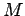and lengthwhich is free to move in one dimension (see Fig. 54). Suppose that electromagnetic radiation of total energyis emitted from one end of the car, propagates along the length of the car, and is then absorbed at the other end. The effective mass of this radiation is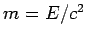(from Einstein's famous relation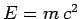). At first sight, the process described above appears to cause the centre of mass of the system to spontaneously shift. This violates the law of momentum conservation (assuming the railway car is subject to no external forces). The only way in which the centre of mass of the system can remain stationary is if the railway car moves in the opposite direction to the direction of propagation of the radiation. In fact, if the car moves by a distancethen the centre of mass of the system is the same before and after the radiation pulse provided that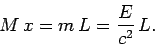(1044)

It is assumed that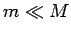in this derivation.

But, what actually causes the car to move? If the radiation possesses momentum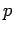then the car will recoil with the same momentum as the radiation is emitted. When the radiation hits the other end of the car then the car acquires momentumin the opposite direction, which stops the motion. The time of flight of the radiation is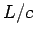. So, the distance traveled by a masswith momentumin this time is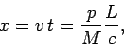(1045)

giving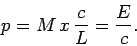(1046)

Thus, the momentum carried by electromagnetic radiation equals its energy divided by the speed of light. The same result can be obtained from the well-known relativistic formula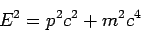(1047)

relating the energy, momentum, and massof a particle. According to quantum theory, electromagnetic radiation is made up of massless particles called photons. Thus,(1048)

for individual photons, so the same must be true of electromagnetic radiation as a whole. If follows from Eq. (1046) that the momentum densityof electromagnetic radiation equals its energy density over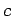, so(1049)

It is reasonable to suppose that the momentum points along the direction of the energy flow (this is obviously the case for photons), so the vector momentum density (which gives the direction, as well as the magnitude, of the momentum per unit volume) of electromagnetic radiation is(1050)

Thus, the momentum density equals the energy flux over.

Of course, the electric field associated with an electromagnetic wave oscillates rapidly, which implies that the previous expressions for the energy density, energy flux, and momentum density of electromagnetic radiation are also rapidly oscillating. It is convenient to average over many periods of the oscillation (this average is denoted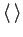). Thus,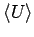(1051)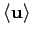(1052)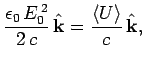(1053)

where the factor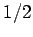comes from averaging. Here,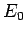is the peak amplitude of the electric field associated with the wave.

Since electromagnetic radiation possesses momentum then it must exert a force on bodies which absorb (or emit) radiation. Suppose that a body is placed in a beam of perfectly collimated radiation, which it absorbs completely. The amount of momentum absorbed per unit time, per unit cross-sectional area, is simply the amount of momentum contained in a volume of lengthand unit cross-sectional area: i.e.,times the momentum density. An absorbed momentum per unit time, per unit area, is equivalent to a pressure. In other words, the radiation exerts a pressure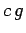on the body. Thus, the radiation pressure is given by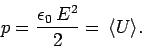(1054)

So, the pressure exerted by collimated electromagnetic radiation is equal to its average energy density.

Consider a cavity filled with electromagnetic radiation. What is the radiation pressure exerted on the walls? In this situation, the radiation propagates in all directions with equal probability. Consider radiation propagating at an angleto the local normal to the wall. The amount of such radiation hitting the wall per unit time, per unit area, is proportional to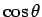. Moreover, the component of momentum normal to the wall which the radiation carries is also proportional to. Thus, the pressure exerted on the wall is the same as in Eq. (1054), except that it is weighted by the average ofover all solid angles, in order to take into account the fact that obliquely propagating radiation exerts a pressure which istimes that of normal radiation. The average ofover all solid angles is, so for isotropic radiation(1055)

Clearly, the pressure exerted by isotropic radiation is one third of its average energy density.

The power incident on the surface of the Earth due to radiation emitted by the Sun is aboutWm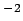. So, what is the radiation pressure? Since,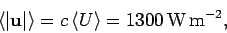(1056)

then(1057)

Here, the radiation is assumed to be perfectly collimated. Thus, the radiation pressure exerted on the Earth is minuscule (one atmosphere equals about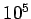Nm). Nevertheless, this small pressure due to radiation is important in outer space, since it is responsible for continuously sweeping dust particles out of the Solar System. It is quite common for comets to exhibit two separate tails. One (called the gas tail) consists of ionized gas, and is swept along by the solar wind (a stream of charged particles and magnetic field-lines emitted by the Sun). The other (called the dust tail) consists of uncharged dust particles, and is swept radially outward from the Sun by radiation pressure. Two separate tails are observed if the local direction of the solar wind is not radially outward from the Sun (which is quite often the case).

The radiation pressure from sunlight is very weak. However, that produced by laser beams can be enormous (far higher than any conventional pressure which has ever been produced in a laboratory). For instance, the lasers used in Inertial Confinement Fusion (e.g., the NOVA experiment in Lawrence Livermore National Laboratory) typically have energy fluxes of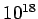Wm. This translates to a radiation pressure of about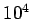atmospheres!Next: Momentum conservation Up: Electromagnetic energy and momentum Previous: Energy conservation
Richard Fitzpatrick 2006-02-02